Smith Chart Basics

Click here to go to a page on plotting Smith Charts with Excel

The Smith chart was developed by Philip Smith at Bell Telephone's Radio Research Lab during the 1930s. Be sure to check out our entry on Philip Smith in our Microwave Hall of Fame! Phil's widow until recently operated Analog Instruments, the company that sold the official Smith chart for many, many years. The trademark on the Smith Chart recently expired, and Ms. Smith sold the Copyright for the Smith Chart to the IEEE MTT Society in April 2015.   Dr. Richard Snyder participated in the negotiations with Ms. Smith and her family. You may notice from time to time that the Smith chart is used in the background on presentations at MTT-S venues.

New for December 2018! The first year ECE students under the guidance of Dr S Raghavan, Professor, ECE dept., N I T Trichy, came out for the first time with the novel idea of putting a Smith chart on ...wait for it... a CAKE! We think it looks delicious.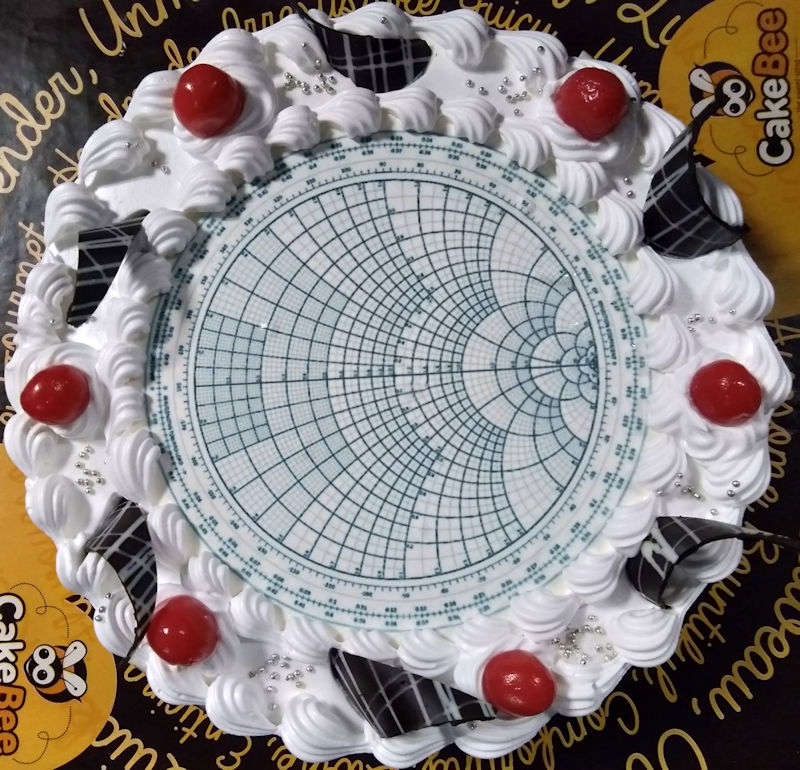We've got our own Smith chart tutorial here, thanks to a fan from Florida, Mike Weinstein, who really knows this subject, and is a fine writer too. If anyone else wants to be a technical contributor on their favorite microwave subject, please contact us.

A clickable index to our Smith chart page:

What's a Smith Chart?

Which way is up and where's that short circuit?

"Yes sir!" and please don't flip me!

Normalization

How much is that stub worth?

Single-stub matching

What's a Smith chart?

What is a Smith chart? It's really just a plot of complex reflection overlaid with an impedance and/or admittance grid referenced to a 1-ohm characteristic impedance. That's it! Transmission coefficient, which equals unity plus reflection coefficient, may also be plotted (see below). You can find books and articles describing how a Smith chart is a graphical representation of the transmission line equations and the mathematical reasons for the circles and arcs, but these things don't really matter when you need to get the job done. What matters is knowing the basics and how to use them, like always.

The Smith chart contains almost all possible impedances, real or imaginary, within one circle. All imaginary impedances from - infinity to + infinity are represented, but only positive real impedances appear on the "classic" Smith chart. Yes, it is possible to go outside the Smith chart "unity" circle, but only with an active device because this implies negative resistance.

One thing you give up when plotting reflection coefficients on a Smith chart is a direct reading of a frequency axis. Typically, plots that are done over any frequency band have markers calling out specific frequencies.

Why use a Smith chart?  It's got all those funny circles and arcs, and good ol' rectangular plots are much better for displaying things like VSWR, transmission loss, and phase, right? Perhaps sometimes a rectangular plot is better, but a Smith chart is the RF engineer's best friend! It's easy to master, and it adds an air of "analog coolness" to presentations, which will impress your friends, if not your dates! A master in the art of Smith-charting can look at a thoroughly messed up VSWR of a component or network, and synthesize two or three simple networks that will impedance-match the circuit in his head!

A quick refresher on the basic quantities that have units of ohms or its reciprocal, Siemens (sometimes called by its former name, mhos), is helpful since many of them will be referenced below. We all think of resistance (R) as the most fundamental of these quantities, a measure of the opposition to current flow that causes a potential drop, or voltage, according to Ohms Law: V=I*R. By extension, impedance (Z) is the steady state AC term for the combined effect of both resistance and reactance (X), where Z=R+jX. (X=ωL for an inductor, and X=1/ωC for a capacitor, where ω is the radian frequency or 2*pi*f.) Generally, Z is a complex quantity having a real part (resistance) and an imaginary part (reactance).

We often think in terms of impedance and its constituent quantities of resistance and reactance. These three terms represent "opposition" quantities and are a natural fit for series-connected circuits where impedances add together. However, many circuits have elements connected in parallel or "shunt" that are a natural fit for the "acceptance" quantity of admittance (Y) and its constituent quantities of conductance (G) and susceptance (B), where Y=G+jB. (B=ωC for a capacitor, and B=1/ωL for an inductor.) Admittances add together for shunt-connected circuits. Remember that Y=1/Z=1/(R+jX), so that G=1/R only if X=0, and B=-1/X only if R=0.

When working with a series-connected circuit or inserting elements in series with an existing circuit or transmission line, the resistance and reactance components are easily manipulated on the "impedance" Smith chart. Similarly, when working with a parallel-connected circuit or inserting elements in parallel with an existing circuit or transmission line, the conductance and susceptance components are easily manipulated on the "admittance" Smith chart. The "immittance" Smith chart simply has both the impedance and admittance grids on the same chart, which is useful for cascading series-connected with parallel-connected circuits.

Which way is up and where's that short circuit?

The most common orientation of the Smith chart places the resistance axis horizontally with the short circuit (SC) location at the far left. There's a good reason for this: the voltage of the reflected wave at a short circuit must cancel the voltage of the incident wave so that zero potential exists across the short circuit. In other words, the voltage reflection coefficient must be -1 or a magnitude of 1 at an angle of 180 degrees. Since angles are measured from the positive real axis and the real axis is horizontal, the short circuit location and horizontal orientation make sense. ("Voltage" is underlined above because the current reflection coefficient of a short circuit being +1 would place the short circuit location at the right end, but let's not go there.)

For an open circuit (OC), the reflected voltage is equal to and in phase with the incident voltage (reflection coefficient of +1) so that the open circuit location is on the right. In general, the reflection coefficient has a magnitude other than unity and is complex. For reasons we won't bore you with here, anywhere above the real axis is inductive (L) and anywhere below is capacitive (C).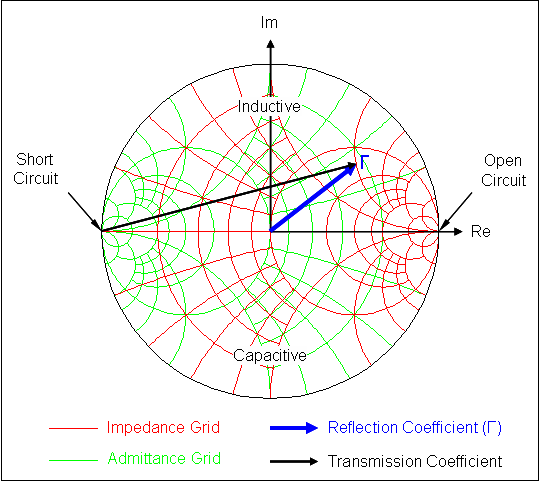"Yes sir!" and please don't flip me!

Can't remember which way to rotate the locus when moving along the transmission line?  Well, it's clockwise toward the generator because generals make you go like clockwork. Also keep in mind that moving "x" degrees along the line moves a point on the locus "2x" degrees on the chart because the reflected wave must transverse the round-trip distance moved (remember, it's the reflection coefficient). Alternately, you could remember that the impedance repeats itself every half wavelength along a uniform transmission line, so you must move one time around the chart to wind up at the same impedance. Of course, a physical line length has variable electrical length over a frequency band, so a fixed impedance will spread out to a locus when viewed through a connected transmission line. This is why it is always easier to obtain a wideband match when you're close to the device or discontinuity.

Many older RF engineers advocate reflecting through the origin to "convert" from impedance to admittance and vice versa. That's why you see the same axis labeled "INDUCTIVE REACTANCE OR CAPACITIVE SUSCEPTANCE" on the original Smith chart, for example. This can be confusing, you've got to do the flip, you need to remember what the grid currently represents, and SC, OC, L & C are moving targets! Why not just keep the reflection coefficient where it belongs and use the appropriate grid? We have computers, color printers, and immittance charts these days. (If you still like to do things manually and either can't deal with all those lines on an immittance chart or are color blind, use a transparency overlay and a blank piece of paper.)

Normalization

Moving along a uniform transmission line doesn't change the magnitude of the reflection coefficient or its radial distance plotted on the Smith chart. But what about when the impedance of the line changes, for example, when a quarter-wavelength transformer is used? Reflection coefficient (Gamma) is, by definition, normalized to the characteristic impedance (Z0) of the transmission line:

Gamma = (ZL-Z0) / (ZL+Z0)

where ZL is the load impedance or the impedance at the reference plane. Note that Gamma is generally complex. Likewise, the impedance (admittance) values indicated on the grid lines are normalized to the characteristic impedance (admittance) of the transmission line to which the reflection coefficient is normalized.

When Z0 changes just past the junction between two different transmission lines, so does the reflection coefficient. Determining the new impedance (admittance) is simple: multiply by the characteristic impedance (admittance) of the current line (this yields the unnormalized value), then divide by the characteristic impedance (admittance) of the new line to obtain the new renormalized value.

The new Gamma may be calculated with the formula above or graphically determined by drawing a line from the origin to the new renormalized value. This example ignores the effect of the step discontinuity encountered in physical (non-ideal) transmission lines, which typically introduces some shunt capacitance.

How much is that stub worth?

Transmission line stubs are essential for impedance matching, introducing small amounts of phase delay (in pairs to cancel reflections), biasing, etc. Are you sometimes unsure that a short-circuited stub that's less than a quarter wavelength is inductive, or whether a wide, low impedance stub in shunt with the main line has low or high Q? A smith chart can tell you these things and give you hard numbers in a jiffy.

For example, a short-circuited stub is just a short circuit seen through a length of transmission line. Place your pencil at the SC point on the chart and move clockwise toward the generator (at the other end of the stub) on the rim by an amount less than a quarter wavelength (180 degrees on the chart). This is in the inductive region; moving more than 180 degrees makes the stub input look capacitive. At exactly one-quarter wavelength, the impedance is infinite, an open circuit. You can do the same for an open-circuited stub by starting at the OC point on the chart.

The real power of the Smith chart comes into play for analysis over a frequency band. Suppose you want to know the susceptance variation of a 50-ohm short-circuited stub over a 3:1 band. This stub could be placed in shunt with the main line at the proper point to double-tune a series-resonant locus, for instance. (We'll cover double-tuning, a very powerful technique, in a future update.) Shown in the admittance chart below is a short-circuited stub that's one-eight wavelength long at the low end and thus is three-eighths wavelengths long at the high end of the 3:1 frequency band. The normalized susceptance varies from -1.0 siemens (inductive) at flow to zero (open circuit) at midband to +1.0 siemens (capacitive) at fhigh. Therefore, the unnormalized susceptance varies between Â±1.0*Y0 siemens, where Y0 (=1/Z0) is the characteristic admittance of the stub. When the characteristic admittance (Y0) of the stub is the same as the main line, the normalized susceptance of the stub may be added to the normalized admittance of the load at each frequency to yield the normalized admittance of the parallel combination. When Y0 of the stub differs from that of the main line, renormalize the stub's susceptance by Y0 of the main line before adding.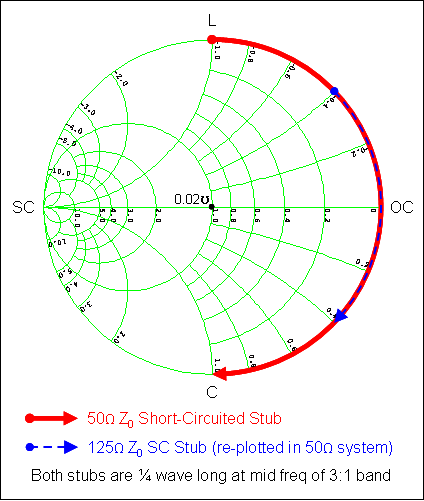Generally, the desired susceptance variation is other than Â±0.02 siemens (Â±1.0*Y0), which a 50-ohm stub would provide in this example. Suppose a 50-ohm main line locus needs a normalized susceptance variation of only Â±0.4 siemens instead of Â±1.0 siemens. Achieve this simply by making the characteristic admittance of the stub equal to 0.4 times that of the main line or Y0=0.4*0.02=0.008 siemens. The stub is now a 125-ohm line (50/0.4) and its susceptance varies less over the band, so it has lower Q. Note that the unnormalized values are rarely needed, normalized values may be renormalized by the ratio of the characteristic impedances involved.

Next, consider a stub for changing the transmission phase of a main-line signal. We know that an open-circuited stub less than a quarter wavelength long retards the phase (adds phase delay), and this is readily seen on the Smith chart: Moving clockwise from the OC position, an open-circuited stub has a transmission coefficient (1 + Gamma) with a negative phase angle. Similarly, a short-circuited stub less than a quarter wavelength long will advance the phase. The following figure illustrates the phase delay of 50-ohm and 25-ohm open-circuited stubs in shunt with a 50-ohm main line. Note that the result is mismatched, which is why stubs should be added in pairs to cancel reflections. Also note that the amount of phase delay increases as the characteristic impedance of the stub decreases (a larger Y0 produces a larger unnormalized susceptance), which makes sense since a wider stub looks like a larger capacitor.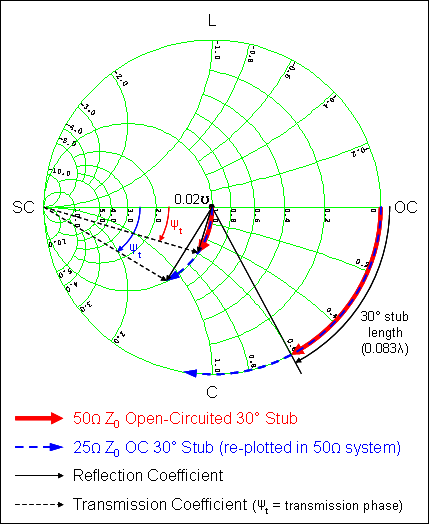Single-stub matching

The ability to obtain a reasonable match over a frequency band depends upon the magnitude of the mismatch, the desired bandwidth, and the complexity of matching circuit. But at any one frequency any impedance mismatch can be perfectly matched to the characteristic impedance of the transmission line, as long as it's not on the rim of the chart (perfect reflection, |Gamma| = 1). And this always can be done with one stub that's less than a quarter-wavelength long. The technique is simple: move along the transmission line to rotate the mismatch to the unity resistance (conductance) circle and insert the appropriate type and length of stub in series (shunt) with the main line to move along this circle to the origin. If the far end of the stub is either a short or open circuit (or generally, any pure reactance), its input end is also a pure reactance (susceptance) so that it doesn't affect the resistance (conductance) component of the mainline impedance (admittance).

Since it's usually easier to add a stub in parallel with a transmission line, the example shown below uses an admittance chart because, at the attachment point, the resulting admittance is the sum of the stub's input susceptance and the main line admittance. First, the mismatched point is rotated around the origin until it reaches the unity conductance circle. Then, the characteristic impedance and length of the stub is chosen such that its input susceptance is equal and opposite to the main line susceptance indicated on the unity conductance circle. The example shows two cases: move toward the generator 39 degrees of line and add a short-circuited stub that provides 0.8 siemens normalized inductive susceptance, or move toward the generator 107 degrees of line and add an open-circuited stub that provides 0.8 siemens normalized capacitive susceptance.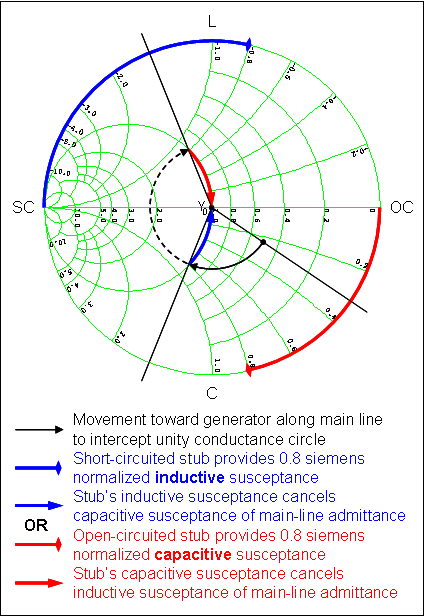There are an infinite number of possible solutions because, at one frequency, a stub of any characteristic impedance can provide the necessary normalized susceptance simply by adjusting its length. The differences show up when looking over a frequency band. For example, the stub's length may be increased by an integer multiple of half-wavelengths at a particular frequency and its input susceptance at this frequency will not change. But over a frequency band, the susceptance will vary considerably more than if the extra length had not been added.

http://www.sss-mag.com/smith.html

Co-Author: Mike Weinstein

Author : Unknown Editor